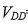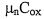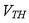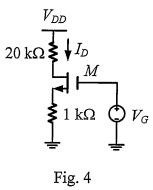# 中山◆電機◆電子學題庫

【非選題】

4. (30%) Figure 4 shows a MOSFET transistor M that makes a constant current source for a 20-kΩ load. The body terminal and source terminal of MOSFET M are tied together. Assume that= 15 V, and the transistor M has the device parameters: WIL = 80,= 50 μA/V2,=0.7 V,λ = 0.1. Suppose we want to have ID = 0.5 mA.【題組】

(c) (10%) Suppose the 15V supply voltage has 1% variations on it (i.e.,△=± 0.15V). Estimate the percentage change in current Io. Notefor M in saturation.